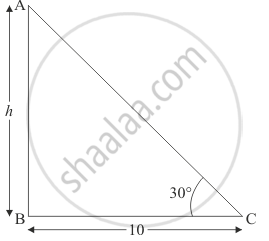Share

# A Tree Breaks Due to the Storm and the Broken Part Bends So that the Top of the Tree Touches the Ground Making an Angle of 30° with the Ground. the Distance from the Foot of the Tree to the Point Where the Top Touches the Ground is 10 Metres. Find the Height of the Tree. - CBSE Class 10 - Mathematics

#### Question

A tree breaks due to the storm and the broken part bends so that the top of the tree touches the ground making an angle of 30° with the ground. The distance from the foot of the tree to the point where the top touches the ground is 10 metres. Find the height of the tree.

#### Solution

Let AB be the tree of height h. And the top of the tree makes an angle of 30° with the ground. The distance between the foot of the tree to the point where the top touches the ground is 10M.

lET bc = 10 And ∠ACB = 30°

Here we have to find the height of the tree.

Here we have the corresponding figureSo we use trigonometric ratios.

In a triangle ABC

=> tan C = (AB)/(BC)

=> tan  30^@ = (AB)/((BC)

=> 1/sqrt3 = h/10

=> h = 10/sqrt3

Now in tiangle ABC we have

sin 30^@ = h/(AC)

=> 1/2 = 10/(sqrt3 AC)

=> AC = 20/sqrt3

So the length of the tree is

 = AB + AC

= h + AC

= 10/sqrt3 + 20/sqrt3

= 10sqrt3

= 1.73

Hence the height of tree is 17.3 m

Is there an error in this question or solution?

#### Video TutorialsVIEW ALL 

Solution A Tree Breaks Due to the Storm and the Broken Part Bends So that the Top of the Tree Touches the Ground Making an Angle of 30° with the Ground. the Distance from the Foot of the Tree to the Point Where the Top Touches the Ground is 10 Metres. Find the Height of the Tree. Concept: Heights and Distances.
S